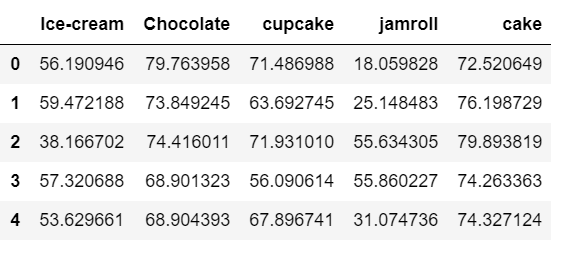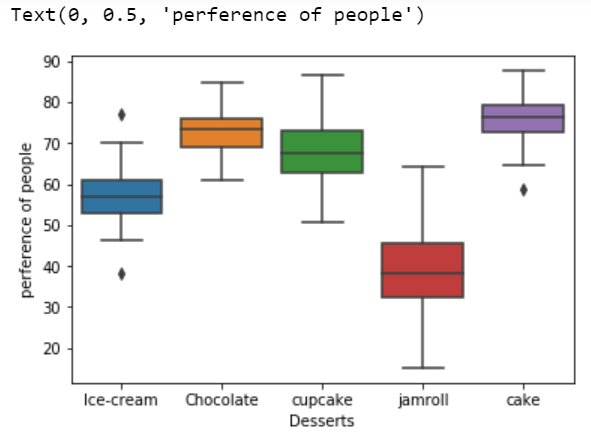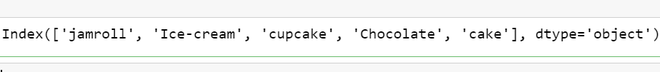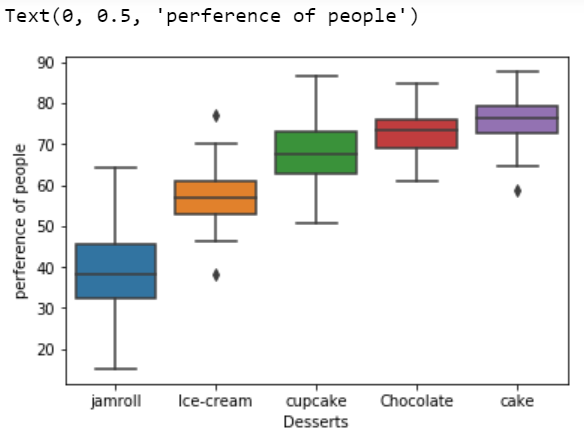Related Articles

# Sort Boxplot by Mean with Seaborn in Python

• Last Updated : 26 Nov, 2020

Seaborn is an amazing visualization library for statistical graphics plotting in Python. It provides beautiful default styles and color palettes to make statistical plots more attractive. It is built on the top of matplotlib library and also closely integrated to the data structures from pandas.
Box Plot is the visual representation of the depicting groups of numerical data through their quartiles. Boxplot is also used for detecting the outlier in data set. It captures the summary of the data efficiently with a simple box and whiskers and allows us to compare easily across groups. Boxplot summarizes sample data using 25th, 50th, and 75th percentiles. These percentiles are also known as the lower quartile, median and upper quartile.

Sometimes, we want to order the boxplots according to our needs there are many ways you can order a boxplot that are:

• Order of boxplot manually
• Sorting of boxplot using mean

In this article, we will discuss how to order a boxplot using mean.

### What sort boxplot using mean?

When we have multiple groups it’s suggested to use sorting by mean or median manually it will get difficult to sort.

#### Step-by-step Approach:

• Importing Libraries

## Python3

 `# import required modules``import` `numpy as np``import` `pandas as pd``import` `seaborn as sns``import` `matplotlib.pyplot as plt`
• Creating dataset

## Python3

 `# creating dataset``df ``=` `pd.DataFrame({``    ``'Ice-cream'``: np.random.normal(``57``, ``5``, ``100``),``    ``'Chocolate'``: np.random.normal(``73``, ``5``, ``100``),``    ``'cupcake'``: np.random.normal(``68``, ``8``, ``100``),``    ``'jamroll'``: np.random.normal(``37``, ``10``, ``100``),``    ``'cake'``: np.random.normal(``76``, ``5``, ``100``),`` ` `})``df.head()`

Output:• Plot the data before sorting the boxplot.

## Python3

 `# plot th data into boxplot`` ` `sns.boxplot(data``=``df)`` ` `# Label x-axis``plt.xlabel(``'Desserts'``)`` ` `# labels y-axis``plt.ylabel(``'perference of people'``)`

Output:• Now sort the data first and get the sorted indices as we have to  sort the boxplot using mean, so we will apply the mean() and sort_values function to the data.

## Python3

 `# This will give the indices of the sorted ``# values into the ascending order the default ``# value in sort_values is ascending = True``index_sort ``=` `df.mean().sort_values().index``index_sort`

Output:• Using sorted index we can sort the data frame that we created.

## Python3

 `# now applying the sorted ``# indices to the data``df_sorted ``=` `df[index_sort]`

So We have sorted the data let’s plot the boxplot of the data.

## Python3

 `# plotting the boxplot for the data ``sns.boxplot(data ``=` `df_sorted)`` ` `# Label x-axis``plt.xlabel(``'Desserts'``)`` ` `# labels y-axis``plt.ylabel(``'perference of people'``)`

Output:If one wants to sort in descending order then use the below syntax:

```index_sort = df.mean().sort_values(ascending=False).index
```

Below is the complete program based on the above approach:

## Python3

 `# import required modules``import` `numpy as np``import` `pandas as pd``import` `seaborn as sns``import` `matplotlib.pyplot as plt`` ` ` ` `# creating dataset``df ``=` `pd.DataFrame({``    ``'Ice-cream'``: np.random.normal(``57``, ``5``, ``100``),``    ``'Chocolate'``: np.random.normal(``73``, ``5``, ``100``),``    ``'cupcake'``: np.random.normal(``68``, ``8``, ``100``),``    ``'jamroll'``: np.random.normal(``37``, ``10``, ``100``),``    ``'cake'``: np.random.normal(``76``, ``5``, ``100``),`` ` `})`` ` ` ` `# sort on the basis of mean``index_sort ``=` `df.mean().sort_values().index`` ` `# now applying the sorted indices to the data``df_sorted ``=` `df[index_sort]`` ` ` ` `# plotting the boxplot for the data ``sns.boxplot(data ``=` `df_sorted)`` ` `# Label x-axis``plt.xlabel(``'Desserts'``)`` ` `# labels y-axis``plt.ylabel(``'perference of people'``)`

Output:Attention geek! Strengthen your foundations with the Python Programming Foundation Course and learn the basics.

To begin with, your interview preparations Enhance your Data Structures concepts with the Python DS Course. And to begin with your Machine Learning Journey, join the Machine Learning – Basic Level Course

My Personal Notes arrow_drop_up